# g(r)

### 为什么是密度？

0K 时固体 g(r)250K 时液体 g(r)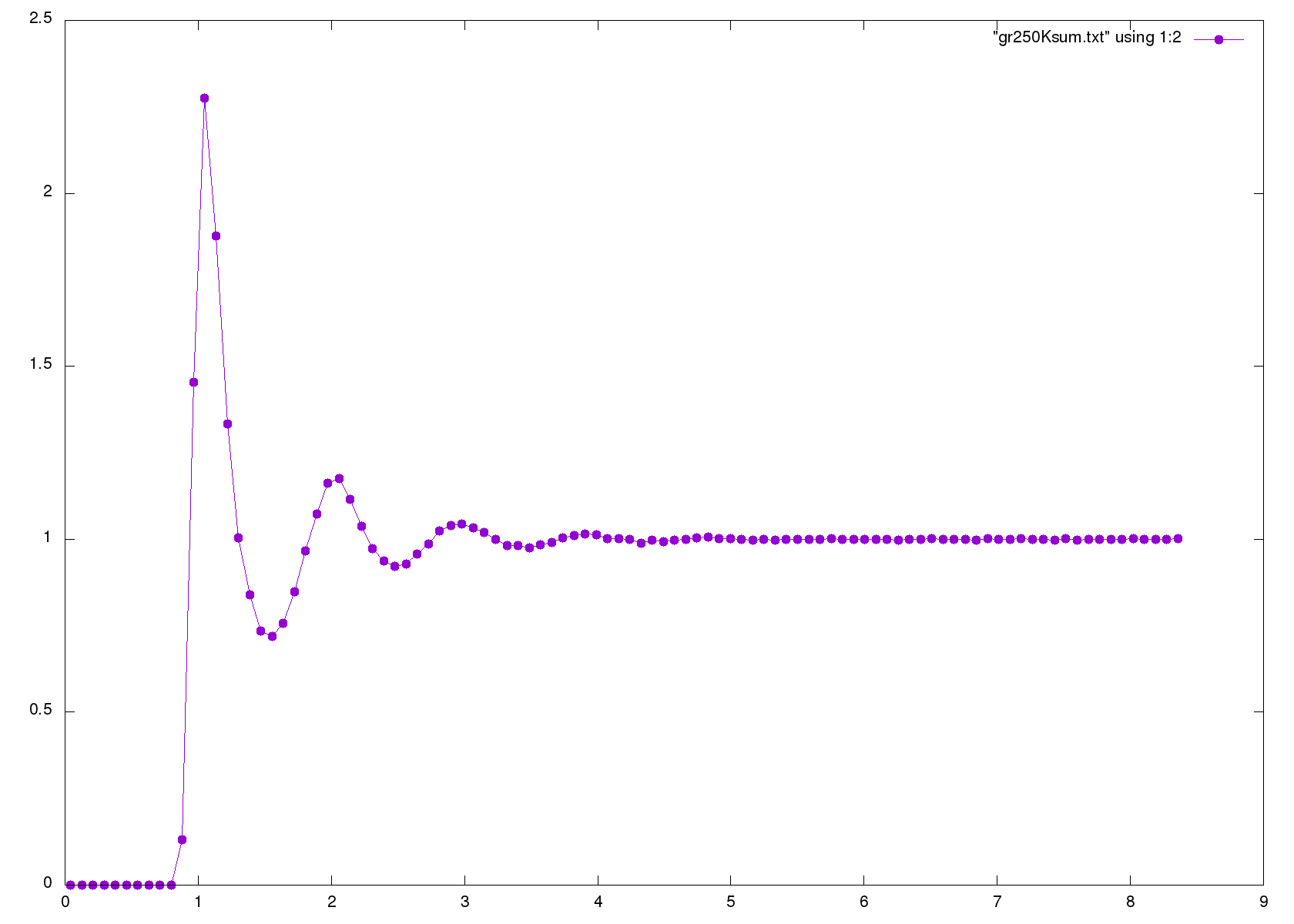# MSD

${\rm MSD}\equiv\langle (x-x_0)^2\rangle=\frac{1}{N}\sum_{n=1}^N (x_n(t) - x_n(0))^2$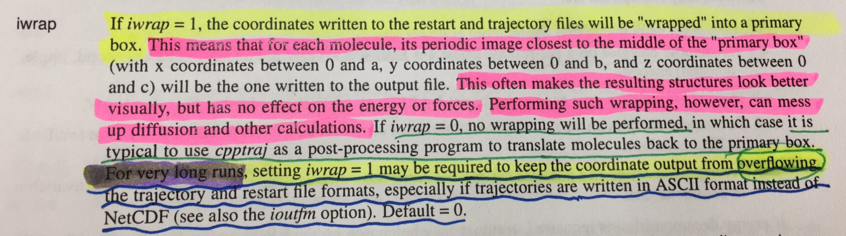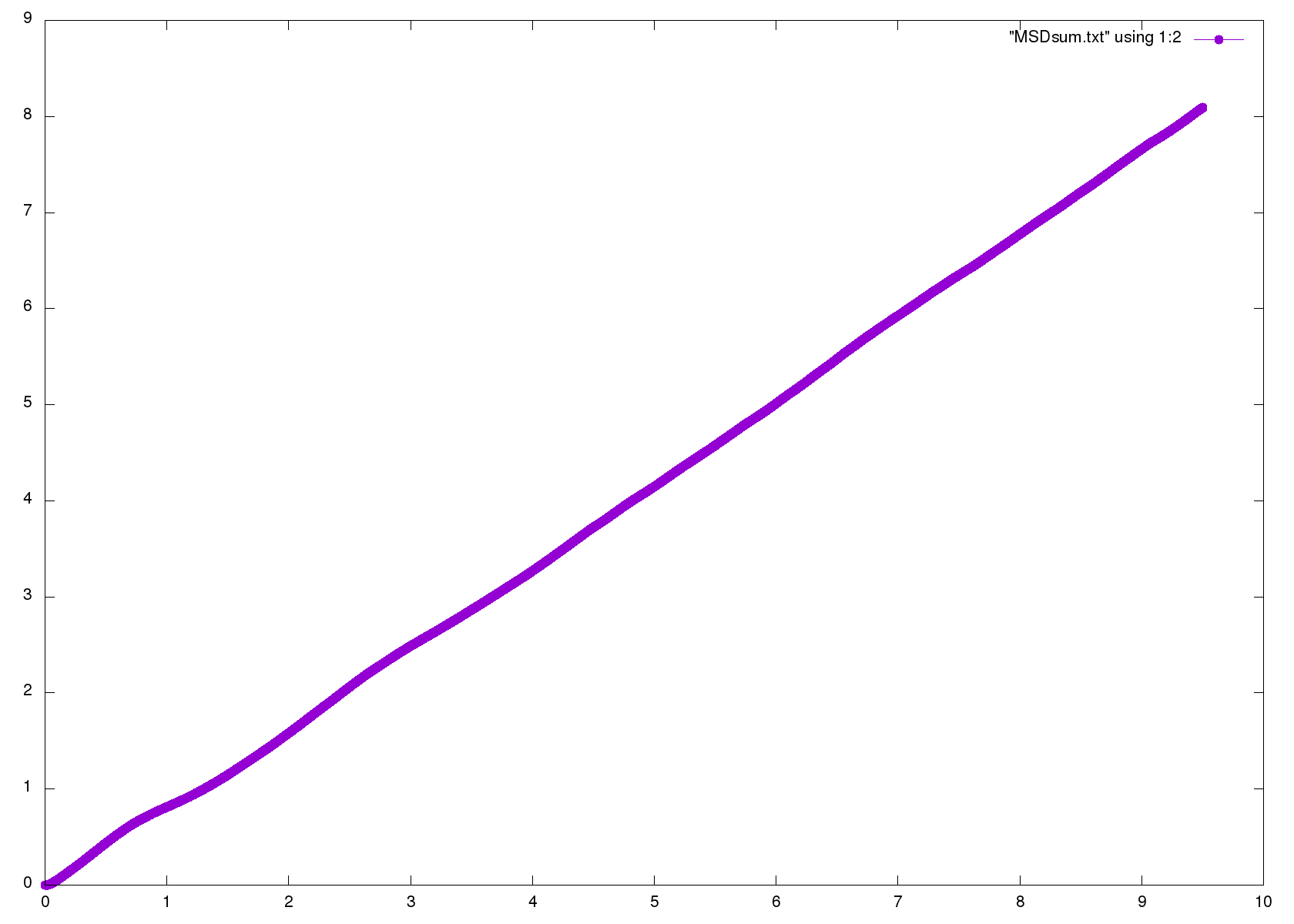# VAF

VAF就是velocity autocorrelation function (VAF) ，是关于速度的自相关函数。自相关函数是表达体系内在关联性质的重要函数。

VAF 图像如下: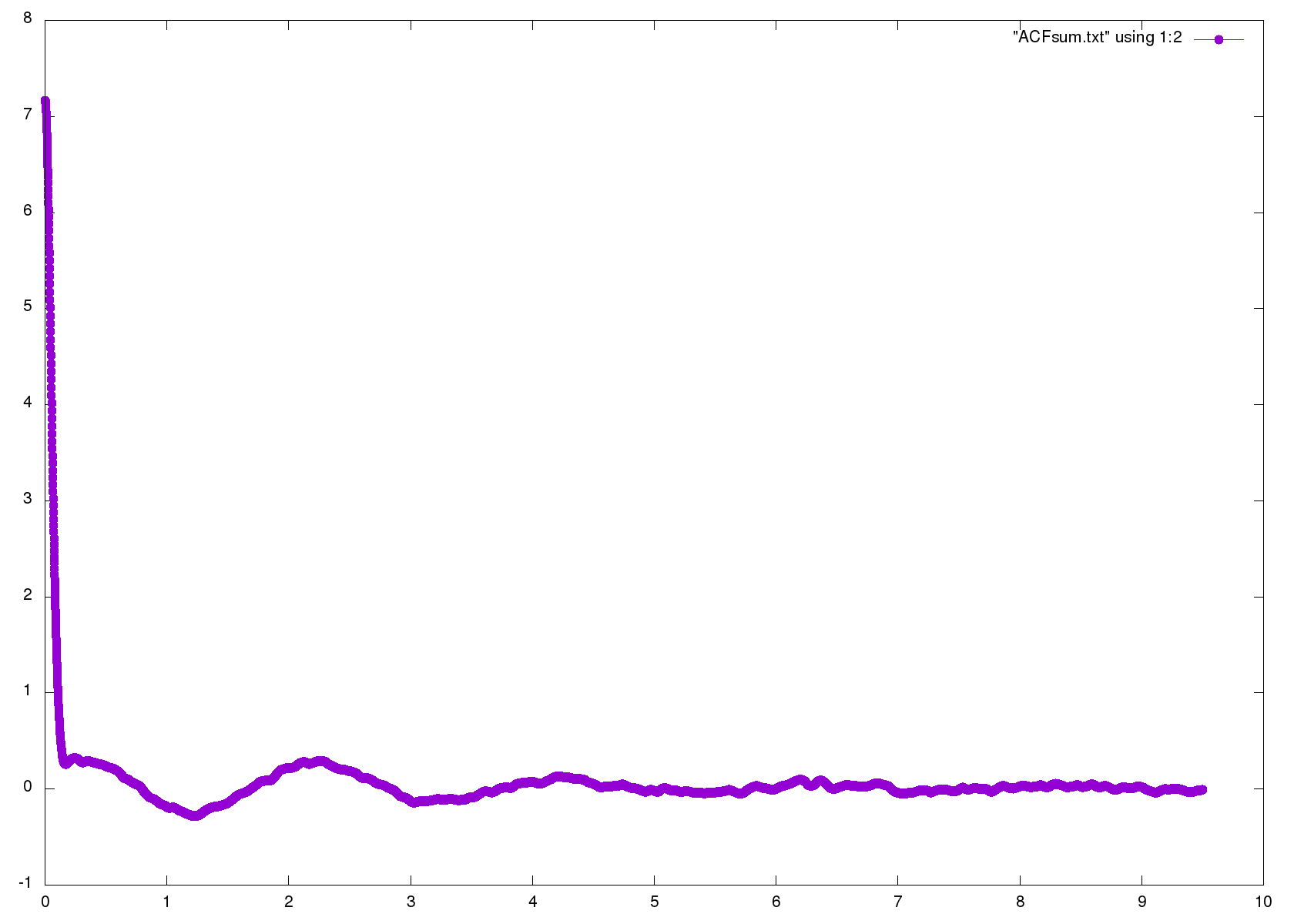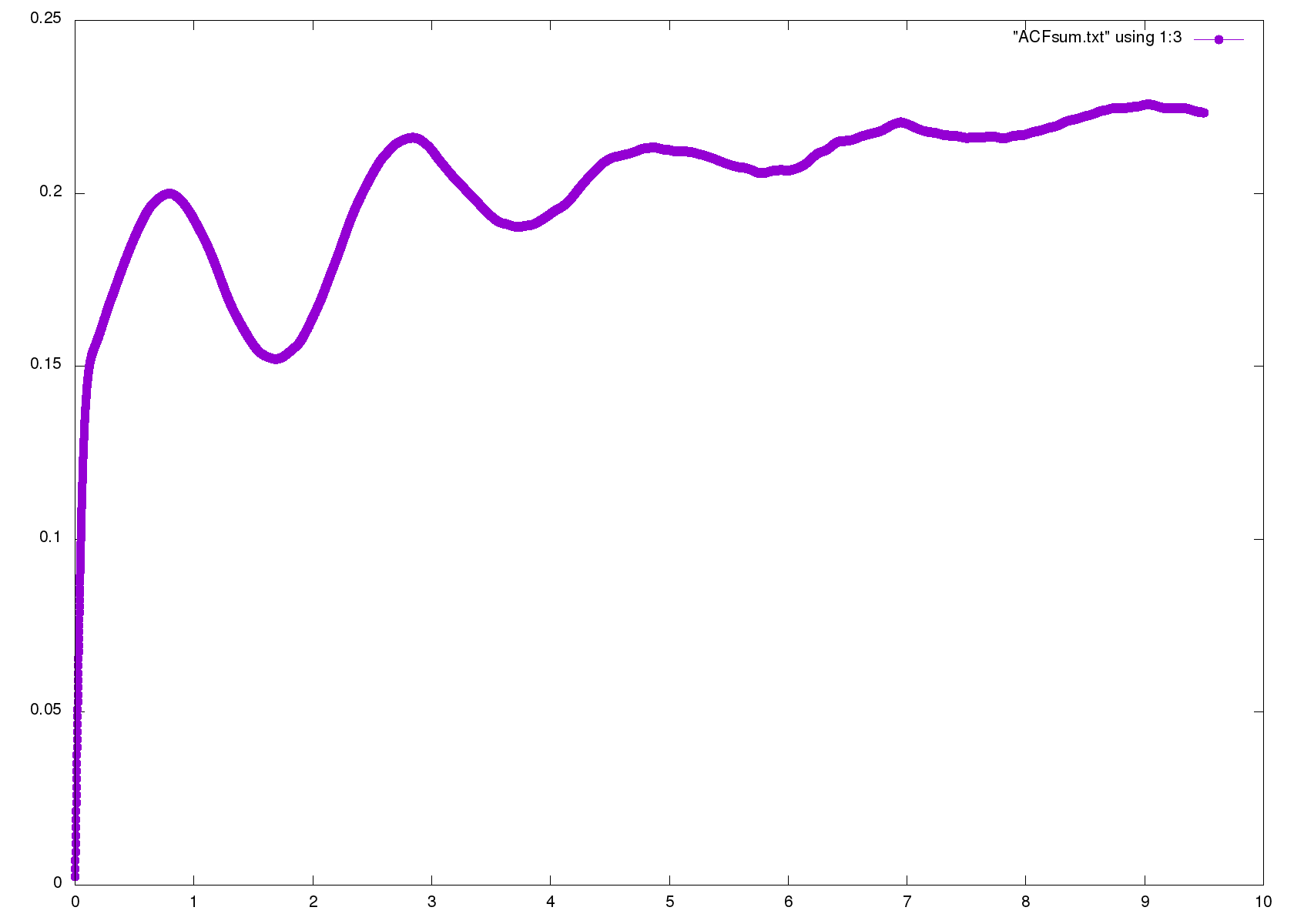Written on March 30, 2017